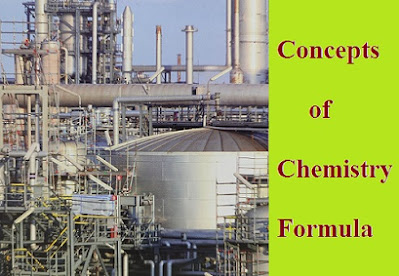# Basic Concepts of Chemistry and Formula

Chemistry is the scientific study of matter and its properties, composition, structure, and the changes that occur during chemical reactions.

It provides the foundation for understanding the world around us, from the structure of matter to the interactions between different elements. Here are some basic concepts.

### Percentage Yield Formula

Actual
Percentage  Yield = -------------------------
Theoretical yield x 100

Mass of one atom of the element

### Atomic mass formula

Atomic mass = --------------------------------------  = Eq. mass X valency
1/12 Mass of an atom of C-12

Eq. mass x Valency                           6.4
Alomic  mass       =    ------------------------------------ =   ---------------------
Atomicity Specific heat (cal/g)     Specific heat (Cal/g)

Number of gram atoms formula
w(g)
Number of gram atoms = -------------------------------
Gram atomic mass (GAM)

w(g)
Number of gram molecules   = --------------------------
Gram Atomic mass

w(g)
Number of gram equivalents=   --------------------------------
Gram equivalent mass (GEM)

Atomic mass
Equivalent mass of an element = -----------------
ValencyMolecular mass of acid
Equivalent mass of an acid = -------------------------
Basicity

Molecular  mass of base
Equivalent mass of a base = ------------------------------
Acidity

Mass of the element
Percentage composition= -------------------------------- *100
Molar mass of compound

Mass of solute

Mass = --------------------------------*100
Mass of solution

### Molarity formula

W * 1000
Molarity = -------------------------
MB* V (in ml)

### Normality formula

WB *1000
Normality = --------------------
EMB* V(in mL)

WB *1000
Molality (m)= --------------------
MB Wa (in g)

### Mole fraction formula

N                     NA
Mole fraction X= ------------- And --------------
NA + NB                         NA +NB

Molecular mass= 2 x Vapour density

where,  WB =Mass of solute, MB= Molecular mass of solute, WA =Mass ol solvent

Number of moles= Molarity * Volume

Number of equivalents =Normality * Volume

Molecular formula= n * Empirical formula

Molecular formula mass
n = -----------------------------------
Empirical formula mass

### Structure of Atom formula

Radius of nucleus (R) = RA1/3

Ro = Constant (= 1.33 x 10 -13 cm)

A= Mass number of atom

Maximum no, of spectral lines produced when an electron

Jumps from n ----> 1 = n(n - 1) / 2

Magnetic moment = (L{n+2)B.M)1/2

Spin angular momentum= ys(s+1)

Spin multiplicity= 2S+ 1

S=Sum of Spin Quantum numbers